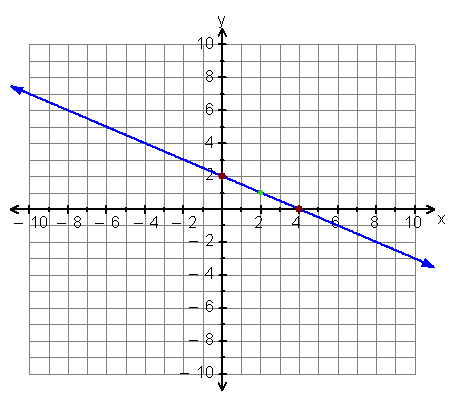# Not able to get the math behind this

I saw a solutions to this problem, but I’m not able to get the logic i.e. why gcd will answer this question ?

Let’s take the example given in the problem:So, here say (x1, y1) = (0, 2) and (x2, y2) = (4, 0)

Now, calculate slope of the line, m = (y2 - y1) / (x2 - x1) = (-2) / 4 = - (1/2)

Now writing the equation of the line,

``````y - y1 = m(x-x1)
or, y - 2 = -(1/2)*(x - 0)
or, y = (4 - x) / 2
Now, for y to be an integer, (4 - x) must be divisible by 2.
That translates to,
4 - x ≡ 0 (mod 2)
or, 4 ≡ x (mod 2)
``````

Now, compare this with the Euclid’s method to find gcd of two numbers and you’ll get your answer!

2 Likes

I came up with a method:

• Lets say the points are (x1,y1) and (x2,y2). Shift the points so that (x1,y1) lies at origin.
• New points: (0,0) and (x2-x1,y2-y1)
• Line joining these two points of form y=mx+c, where m = (y2-y1)/(x2-x1) and c=0
• say x is integer, for y to be integer: ((y2-y1)*x)%(x2-x1) == 0
• take abs(y2-y1) = a, and abs(x2-x1) = b. So now required condition is (a*x)%b == 0.
• Let g = gcd(a,b). Let a1=a/g and b1=b/g.
• now the relation becomes (a1xg)%(b1*g) == 0 where a1 & b1 are co-prime.
• Problem can now be stated as number of x such that (a1*x)%b1 == 0 where a1,b1 and x are defined above.
• Lets say range of x is [0,R] where R = abs(x2-x1), 0 and R inclusive
• Number of x would be multiples of b1 in range of x. This is because a1 and b1 have no common factors other than 1, so for the modulo to become 0 there should be a multiple of b1 in numerator.
• So number of multiples would be floor(R/b1) + 1.(Extra 1 is for 0).Now subtract the two end points and ans becomes floor(R/b1) - 1. Substitute the values and it becomes floor(abs(x2-x1)/(b/g)) - 1 and b is abs(x2-x1).
• After cancelling from numerator and denominator ans is floor(g) - 1 or g-1.
Putting value of g ans becomes gcd(y2-y1,x2-x1) - 1.
2 Likes

I appreciate your answer, but it would be great if it consisted of variables instead of actual values.

I thought it would be better to explain from a layman’s perspective. My bad, Sir! I didn’t know it would have been great that way. Pardon! I thought explaining the example would clear the instinct and you’ll be able to deduce why is it happening… Anyways+1 for the detail

Thanks @ak5hay